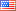##### Modeling and characterization of a photovoltaic array based on actual performance using cascade-forward back propagation artificial neural network.
Publication Type
Original research
Authors
This paper proposes a novel prediction model for photovoltaic (PV) system output current. The proposed model is based on cascade-forward back propagation artificial neural network (CFNN) with two inputs and one output. The inputs are solar radiation and ambient temperature, while the output is output current. Two years of experimental data for a 1.4 kWp PV system are utilized in this research. The monitored performance is recorded every 2 s in order to consider the uncertainty of the system’s output current. A comparison between the proposed model and other empirical and statistical models is done in this paper as well. Moreover, the ability of the proposed model to predict performance with high uncertainty rate is validated. Three statistical values are used to evaluate the accuracy of the proposed model, namely, mean absolute percentage error (MAPE), mean bias error (MBE), and root mean square error (RMSE). These values are used to measure the deviation between the actual and the predicted data in order to judge the accuracy of the proposed model. A simple estimation of the deviation between the measured value and the predicted value with respect to the measured value is first given by MAPE. After that, the average deviation of the predicted values from measured data is estimated by MBE in order to indicate the amount of the overestimation/underestimation in the predicted values. Third, the ability of predicting future records is validated by RMSE, which represents the variation of the predicted data around the measured data. Eventually, the percentage of MBE and RMSE is calculated with respect to the average value of the output current so as to present better understating of model’s accuracy. The results show that the MAPE, MBE, and RMSE of the proposed model are 7.08%, −0.21 A (−4.98%), and 0.315 A (7.5%), respectively. In addition to that, the proposed model exceeds the other models in terms of prediction accuracy.
Journal
Title
Solar Energy Engineering, ,
Publisher
ASME
Publisher Country
United States of AmericaIndexing
Thomson Reuters
Impact Factor
1.329
Publication Type
Both (Printed and Online)
Volume
137
Year
2015
Pages
041010 (1-5).